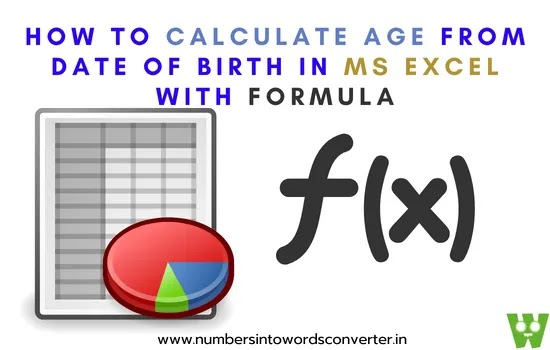# HOW TO CALCULATE AGE FROM DATE OF BIRTH IN EXCEL WITH FORMULA IN 2023

## HOW TO CALCULATE AGE FROM DATE OF BIRTH IN EXCEL WITH FORMULA IN 2023

HOW TO CALCULATE AGE FROM DATE OF BIRTH IN EXCEL | Let's learn what is the formula to calculate age from the date of birth in excel for all versions.HOW TO CALCULATE AGE FROM DATE OF BIRTH IN EXCEL

### WHAT IS THE FORMULA TO CALCULATE AGE FROM DATE OF BIRTH IN EXCEL

Now, Let's learn how to calculate age from date of birth in Microsoft Excel for the windows operating system. I will try to make it simple for you to understand better even if you are a beginner or a student.

Okay, so here I have some names and the detail. So let’s learn how do I calculate age in YYYY MM DD in Excel live step by step.

Alex   12-Jan-86

Joy     02-04-99

Rock  02-05-00

Mariya         09-09-80

Dolli  01-01-85

Raj     03-05-11

Koli   02-05-96

Rahul           06-08-92

Joni   05-05-87

Rani  08-07-80

I want to take the year, month, and date. So it's up to you. If you want to, you can only take Year, or take a Month, or you can take the day.

Okay, so the formula will be the same I will show you below

First, I want to take the year from the date of birth to formalize.

=DATEDIF(SELECT DATE OF BIRTH CELL LOCATION,TODAY(),"Y")

Okay, Use this function =datedif()

If you can't do that if you open the bracket, select the date of birth, insert the comma and write today(), and now open and close the bracket.

Insert the comma double quotation. If you want to take the year just type Y if we want to take the month the type M.

So first we're going to take the quotation and close the quotation. Close the bracket, Finally hit the enter button, and boom.

So we got the Year.

Okay. Now hold the Month. Same thing, just a little tool they gave on bracket select your date of birth.

Insert the comma and type it today open

And close the bracket.

Insert the former or double quotation.

And here I need to type the month, okay simple, and then close the quotation close the docket enter, and boom.

So it will calculate the whole month. So we are not going to take the only month after the years Okay and it will take only a month after the like 36 years, a Total of 437 months. That's it.

If you want to take the entire month you can delete Y it. It will calculate the entire month if you want to take the month after the year.

That's why it will take an Entire month to use M.

That's it. Now if you want to pick the date, type the second formula for calculating the Total Days –

=DATEDIF(SELECT DATE OF BIRTH CELL LOCATION,TODAY(),"MD")

Open the brackets select your date of birth, comma today open-close bracket again comma and now reception, and this time I am the one offered a quotation close the bracket into more details.

Name            Date of Birth          Year  Total Month           Days  Months

Alex   12-Jan-86    36               437         13307                  5

So now you can apply this formula to all the entries. That's it simple. So the formula is simple to calculate what is your live age till now.

If you want to calculate your total months from your date of birth using this formula in any version of Ms Excel.

#### AGE CALCULATOR IN EXCEL FORMULA

=DATEDIF(E5,TODAY(),"M")

If you just want to learn how to know only Days from your birth in Excel then use this function –

=DATEDIF(E5,TODAY(),"D")

If you just want to calculate your months from 1-12 in MS Excel then use this function –

=DATEDIF(E5,TODAY(),"YM")

So this is a simple step to get the year from the date of birth in Excel.

 No. Name Date of Birth Year Total Month Days Months 1 Alex 12-Jan-86 36 437 13307 5 2 Joy 02-04-99 23 278 8479 2 3 Rock 02-05-00 22 265 8083 1 4 Mariya 09-09-80 41 501 15258 9 5 Dolli 01-01-85 37 449 13683 5 6 Raj 03-05-11 11 133 4065 1 7 Koli 02-05-96 26 313 9544 1 8 Rahul 06-08-92 29 358 10909 10 9 Joni 05-05-87 35 421 12829 1 10 Rani 08-07-80 41 503 15321 11

Thanks for reading this article I hope you learned a lot of tricks and tips on how the age calculator formula works in MS Excel worksheet.

There is one factor that always remains the same: the age calculator in Excel formula.

This mathematical tool can be used to determine someone's age with 100% accuracy, no matter what other factors are at play.

IF YOU WANT TO KNOW HOW MANY MINUTES OLD YOU ARE WITH THE FASTEST CALCULATOR THEN I HAVE SHARED THE BEST CALCULATOR ON THIS SITE.

Age calculator logic-

To use the age calculator in Excel formula, simply enter the person's date of birth into a cell. This will output the person's age in years.

You can also use the age calculator in Excel formula to determine the age of something else, like a piece of fruit.

To do this, simply enter the date that the item was created or harvested into a cell, and then type its formula into another cell. This will output the age of the item in years.

Conclude – Here I have explained in detail how to calculate age from date of birth in Microsoft Excel with a formula for all Microsoft Excel versions of the Windows operating system especially 2007, 2010, and 2013.. As well as I have given some best examples too for beginners in 2023.